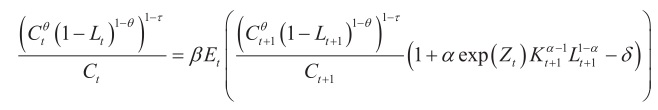# A RBC code problem of determining period

I have a dynamic equation of consumption :and the code in a book is:

# #mar_c1=(c(+1)^theta*(1-lab(+1))^(1-theta))^(1-tau);

mar_c/c=beta*(mar_c1/c(+1))(1+alphaexp(z)*k(-1)^(alpha-1)*lab^(1-
alpha)-delta);
why the variable of K shifts 2 periods and variable lab shifts 1 period? in the equation above, the period of K and lab are both (t+1),

1 Like

I think the code has some problem. Usually the equation in the book looks like:
1+\displaystyle \alpha \exp (Z_{t+1})K_{t+1}^{\alpha-1}L_{t+1}^{1-\alpha}-\delta
But due to the law of motion of capital K_t, we code the equation as if
1+\displaystyle \alpha \exp (Z_{t+1})K_t^{\alpha-1}L_{t+1}^{1-\alpha}-\delta,
that is, K_t is one-period predetermined before L_{t+1}.

But, I don’t understand why 2 periods shift back, as you said. By using auxiliary variables mar_c and mar_c1, the code is now very similar to the basic RBC model, whose dynare code can be found everywhere, and does not shift backwards.

If my saying was wrong, please kindly point it out, thx!1 Like

I found this code from a Chinese author LIXiangyang
this pdf is from his course3.1 a_simple_example.pdf (170.1 KB)

1 Like

Thank you for sharing! It was written in English, great!You can also find its dynare practicing code in Practicing Dynare.pdf (478.5 KB) the first example, neoclassical growth model, originated by Cooley and Prescott (1995), or its updated and expanded version in the forum:

CheersThank you HelloDynare，you are so niceNote that the timing in equation (4) of the screenshot of @HelloDynare is strange and most probably wrong as well.

yeah,I noted that the period is (t+1)